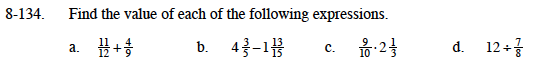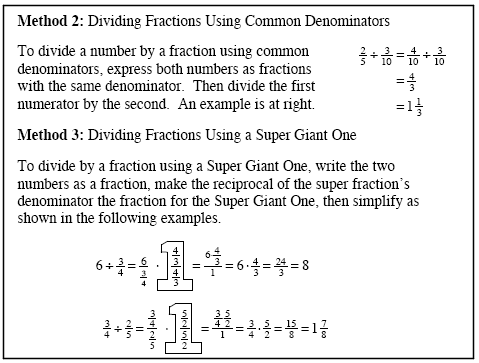### Home > MC1 > Chapter 8 > Lesson 8.4.3 > Problem8-134

8-134.Rewrite the fractions so that they have a common denominator.

Convert these mixed numbers into fractions greater than one, then refer to part (a).

Refer to problem 8-98.

Refer to the Math Notes box below to see two ways of dividing by fractions.

$2\frac{11}{15}$

$13\frac{5}{7}$